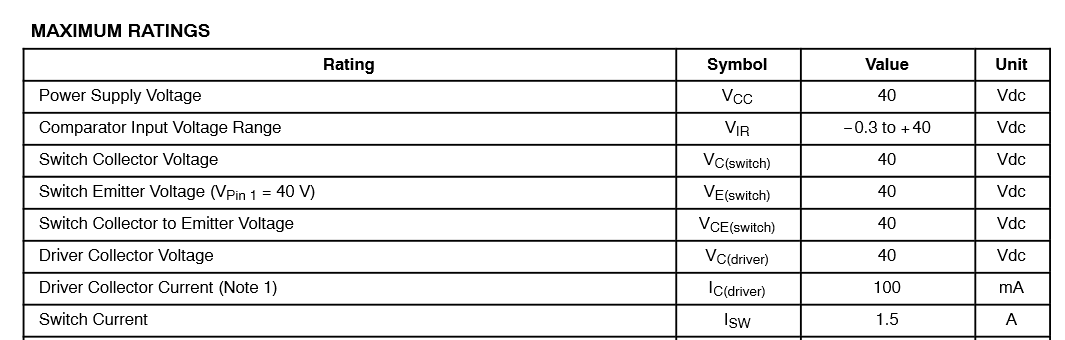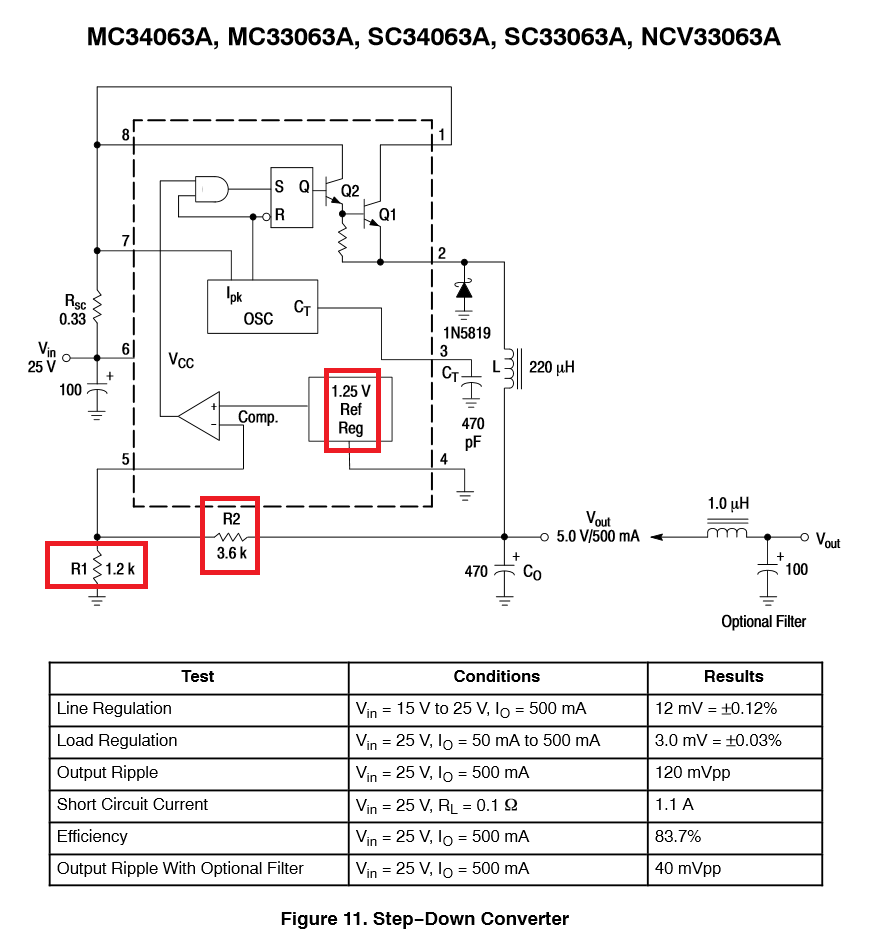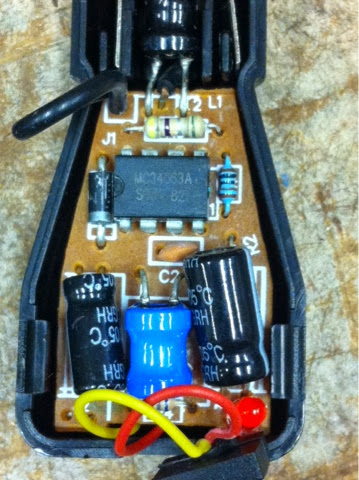## Friday, September 19, 2014

### Change car charger's output voltage

Car charger is a must-have today, thanks to smartphones' larger and larger screen. It is very cheap to get a car charger adapter, which converts 12V, voltage of car's socket, into 5V, voltage of USB standard. I have several of these too.

However, Now I am in need of 9V 1A power while driving, and I decide to convert one of my 5 V car charger into 9 V.

Initially I was thinking about using a 9 V voltage regulator, say, LM7809, to do this. I believe it will work, but I found the solution can be even simpler after I actually opened one 5 V car charger.

This is what it looks like inside the 5 V car charger.

On the top is a 2 A fuse, which connects to the anode of the car charger (12V). The two thin metal sheets connect to the ground. The circuit at the bottom does the 12V-5V converting job.
Although those larger aluminum capacitors at the bottom occupy most of the space, the most crucial part is the IC (integrated circuit) under the black wire.

Look carefully into the circuit I found the IC part number is MC34063A, which is a 1.5A step up/down/invert DC-DC voltage regulator. I found its specs online http://www.onsemi.com/pub_link/Collateral/MC34063A-D.PDFSounds promising. The regulator can theoretically output voltage from 1.25V to 40V, and it can output up to 1.5A. So it is possible to achieve my goal, 9V 1A, by simply modifying the circuit a little bit.

The datasheet also provide a step-down circuit example, which outputs fixed 5V.The output pin of MC34063A is pin 2. Resistor R1 and R2 form a voltage divider so that the voltage of pin 5 is
V5 = Vout*R1/(R1+R2),
which gives
Vout = V5*(R1+R2)/R1 = V5*(1+R2/R1).

From the circuit we see that V5 is tight to a 1.25V reference by a voltage comparator, meaning that V5 must equal to 1.25V. Hence
Vout = 1.25V*(1+R2/R1).

Given R1 = 1.2k and R2 = 3.6k = 3R1, there is
Vout = 1.25V*(1+3) =  5V.Look carefully into the circuit I found that the blue resister on the right side of the IC is labeled R1, and the yellow resistor hidden beneath the large black capacitor is labeled R2. R1 links pin 5 with GND, and R2 links Pin 5 and output (the red wire). They match the above schematics perfectly!

Using a multimeter I found that R1 = 1k ohm and R2 = 3k ohm. Hence Vout = 1.25*(1+3) = 5V.d

Now the task is super easy! I removed R2 and replace it with a 6.2k ohm resistor so that
Vout = 1.25V*(1+6.2k/1k) = 1.25V*7.2=9V.

Guess what, after this simple modification, the voltage output of the car charger is 9V!

I opened several other car chargers I have and they all use MC34063A or identical chips. So looks like it is a pretty standard way to make inexpensive car chargers. Therefore the method I used here would work for those car charges as well.